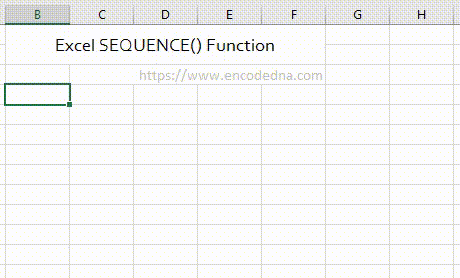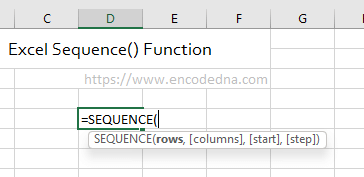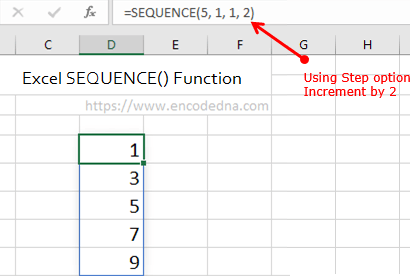﻿ Excel SEQUENCE() Function - How to add Days number quickly in a sequence

# Excel SEQUENCE() Function - How to add Days number quickly in a sequence

Sharing a simple formula in Excel showing how to add days number in sequence quickly. The formula uses the "SEQUENCE()" function, a new built-in function in Excel.

See the below image.### The Formula

`="Days " & SEQUENCE(100, 1, 1)`

The above Excel formula will write days with number in a "sequence" from 1 to 100. So, it wil fill hundred rows with the days number.

## SEQUENCE() Function

The SEQUENCE() returns a sequence of number. Its a newly added function and its only avilable in Microsoft 365, Excel 2021 and Excel for the Web.

### Here's the syntax.The function takes "four" paramters.

1) rows - the number of rows to fill

2) columns - the number of columns to fill. Use this option if you want to fill values in multiple columns.

3) start - the starting number in sequence. This is optional

4) step - the increment between each number in the sequence.🙂